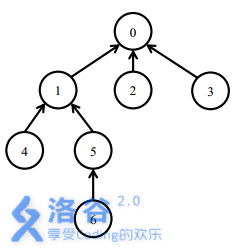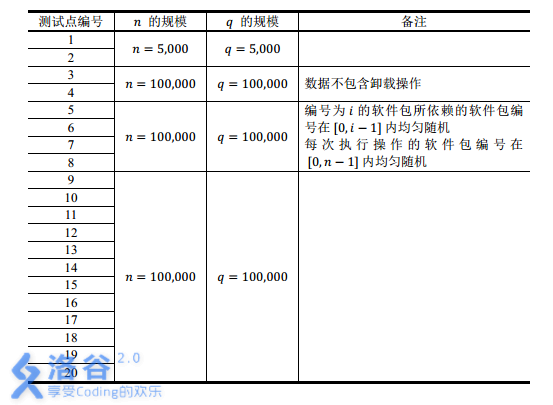# 洛谷 P2146 [NOI2015]软件包管理器 题解【树链剖分】【线段树】

与其说是一道树剖题，不如说是学习了线段树的区间覆盖写法。

## 题目描述

Linux用户和OSX用户一定对软件包管理器不会陌生。通过软件包管理器，你可以通过一行命令安装某一个软件包，然后软件包管理器会帮助你从软件源下载软件包，同时自动解决所有的依赖（即下载安装这个软件包的安装所依赖的其它软件包），完成所有的配置。Debian/Ubuntu使用的apt-get，Fedora/CentOS使用的yum，以及OSX下可用的homebrew都是优秀的软件包管理器。

## 输入输出格式

install x：表示安装软件包x

uninstall x：表示卸载软件包x

## 输入输出样例

7
0 0 0 1 1 5 5
install 5
install 6
uninstall 1
install 4
uninstall 0

3
1
3
2
3

10
0 1 2 1 3 0 0 3 2
10
install 0
install 3
uninstall 2
install 7
install 5
install 9
uninstall 9
install 4
install 1
install 9

1
3
2
1
3
1
1
1
0
1

## 说明

【样例说明 1】【数据范围】比较裸的树链剖分，安装前统计0到要安装软件这条链上的数字和。安装时把这条链全部改为1，这一过程改变的数量为深度d[要安装软件]-之前统计的数字和。

卸载前统计以当前软件为根的子树中数字和，改变的数量就是这个数，卸载时把这棵树上全部改成0。

树剖的思路和板子在这篇文章写了。这里我记一下区间覆盖线段树的做法。

而lazytag+pushdown对于我之前的做法是有弊端的。我之前所写的lazy表示自己没有更新而携带lazytag，而我看网上OIer都写的是携带lazytag的点自己已更新，而pushdown自己的时候更新左右孩子的权值和lazytag，这样条理就清晰了很多。这个题（区间覆盖法）如果这样做的话会看得更清楚，而我原来的做法做这种题就会很麻烦，并且有可能出现多次冗余更新。以后我的线段树习惯应该就改过来了。

## Code：

#include<cstdio>
#include<cstring>
#define ls (k<<1)
#define rs (k<<1|1)
#define mid (l+r>>1)
#define Mid (t[k].l+t[k].r>>1)
struct edge
{
int n;
int nxt;
edge(int n,int nxt)
{
this->n=n;
this->nxt=nxt;
}
edge()
{
nxt=-1;
}
}e;
{
}

struct node
{
int l,r,v,lazy;
node(int l,int r,int v)
{
this->l=l;
this->r=r;
this->v=v;
v=0;
lazy=-1;
}
node(int l,int r)
{
this->l=l;
this->r=r;
v=0;
lazy=-1;
}
node(){}

}t;
void build(int k,int l,int r)
{
t[k]=node(l,r,0);
if(l==r)
return;
build(ls,l,mid);
build(rs,mid+1,r);
}
void pushdown(int k)
{
if(t[k].lazy==-1)
return;
t[k].v=(t[k].r-t[k].l+1)*t[k].lazy;//线段树为“改为”
if(t[k].l==t[k].r)
{
t[k].lazy=-1;
return;
}
t[ls].v=(t[ls].r-t[ls].l+1)*t[k].lazy;
t[ls].lazy=t[k].lazy;
t[rs].v=(t[rs].r-t[rs].l+1)*t[k].lazy;
t[rs].lazy=t[k].lazy;
t[k].lazy=-1;
}
{
pushdown(k);
if(l==t[k].l&&r==t[k].r)
return t[k].v;
if(r<=Mid)
else if(l>Mid)
}
void change(int k,int l,int r,int x)
{
if(t[k].l==l&&t[k].r==r)
{
t[k].v=(r-l+1)*x;
t[k].lazy=x;
//pushdown(k);
return;
}
pushdown(k);
if(r<=Mid)
change(ls,l,r,x);
else if(l>Mid)
change(rs,l,r,x);
else
{
change(ls,l,Mid,x);
change(rs,Mid+1,r,x);
}
t[k].v=t[ls].v+t[rs].v;
return;
}

//dfs求重链，
int d,s,sz;
int fa;
void dfs1(int x,int D)
{
d[x]=D;
sz[x]=1;
s[x]=100001;
{
if(!d[e[i].n])
{
fa[e[i].n]=x;
dfs1(e[i].n,D+1);
sz[x]+=sz[e[i].n];
if(sz[e[i].n]>sz[s[x]])
s[x]=e[i].n;
}
}
return;
}
int dfn,pnt,cnt=0,over;
int top;
void dfs2(int x,int t)
{
dfn[x]=++cnt;
pnt[cnt]=x;
top[x]=t;
if(s[x]!=100001)
dfs2(s[x],t);
{
if(e[i].n==s[x])
continue;
dfs2(e[i].n,e[i].n);
}
over[x]=cnt;
return;
}
int tree(int x)
{
}
void treechange(int x)
{
change(1,dfn[x],over[x],0);//改为0
}
int line(int x,int op)//op=2为修改
{
int y=0,ans=0;
int tx=top[x],ty=top[y];
while(tx!=ty)
{
if(d[tx]<d[ty])//ty深一些
{
if(op==2)
change(1,dfn[ty],dfn[y],1);
else
y=fa[ty];
ty=top[y];
}
else
{
if(op==2)
change(1,dfn[tx],dfn[x],1);
else
x=fa[tx];
tx=top[x];
}
}
if(dfn[x]<dfn[y])
{
if(op==2)
change(1,dfn[x],dfn[y],1);
else
}
else
{
if(op==2)
change(1,dfn[y],dfn[x],1);
else
}
return ans;
}

int main()
{
top=0;
fa=0;
int n,u;
scanf("%d",&n);
build(1,1,n);
for(int i=1;i<n;i++)
{
scanf("%d",&u);
}
dfs1(0,1);
dfs2(0,0);
int m;
scanf("%d",&m);
char c;
while(m--)
{
scanf("%s",c);
if(c=='i')
{
scanf("%d",&u);
int k=line(u,1);
line(u,2);
printf("%d\n",d[u]-k);
}
else
{
scanf("%d",&u);
int k=tree(u);
treechange(u);
printf("%d\n",k);
}
}
return 0;
}


### 说点什么Subscribe

/* */